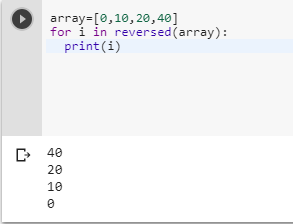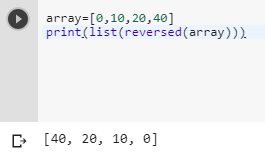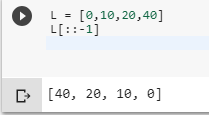2 views
in Python

How can I do the following in Python?

array = [0, 10, 20, 40]

for (i = array.length() - 1; i >= 0; i--)

I need to have the elements of an array, but from the end to the beginning.

by (106k points)
edited by

Actually, to reverse any list in Python we have many ways and many efficient ways than other languages:-

The first thing you can use the reverse function which is in build function to reverse any data types in Python:-

array=[0,10,20,40]

for i in reversed(array):

print(i)Important point to note is, the reversed() function does not return a list. Do get a reversed key you can use list(reversed(array)).

array=[0,10,20,40]

print(list(reversed(array))The second method to do this problem which makes Python unique and best at the work compared to other language is by using the slice method:-

L = [0,10,20,40]

L[::-1]